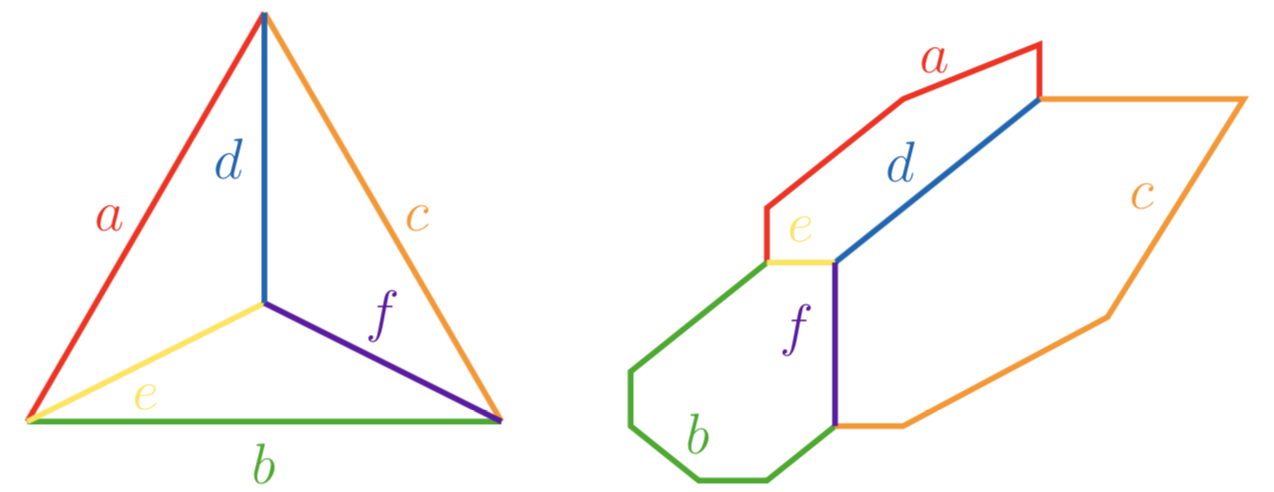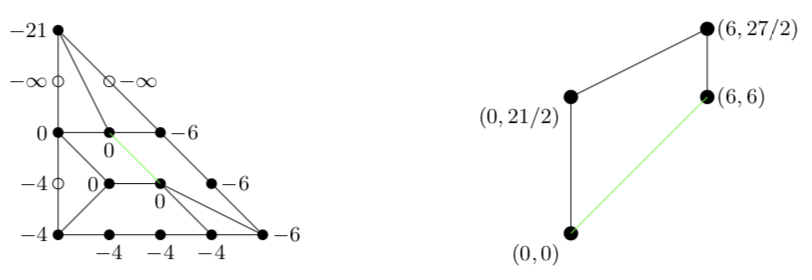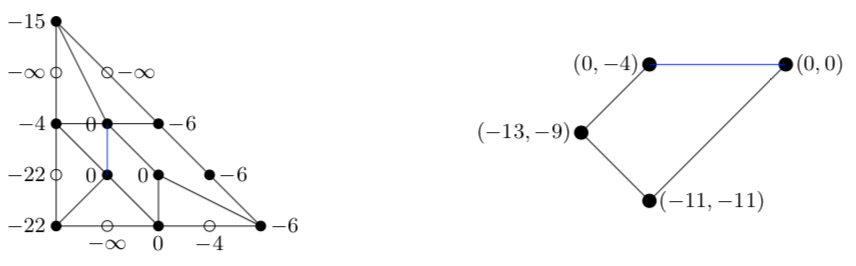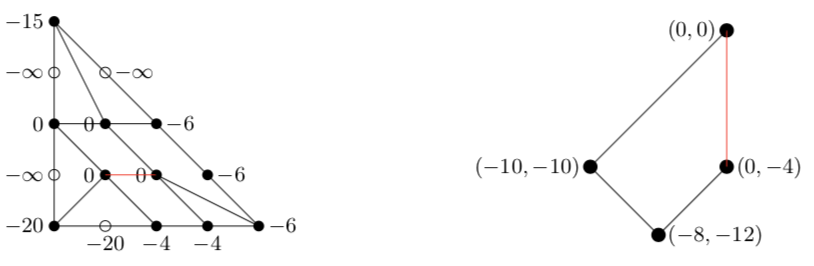# Type (000)¶

## Plane quartics of type (000)¶

A tropical curve $$C$$ of type (000) is of the formDue to symmetry, we may assume that $$b\leq c\leq a$$. It is embeddable as a tropical quartic curve in $$\mathbb{R}^2$$ if $$\max(d,e)\le a$$, $$\max(e,f)\le b$$ and $$\max(d,f)\le c$$, such that

• at most two of the inequalities may hold as equalities,

• if two hold as equalities, then either $$d,e,f$$ are distinct and the edge $$a,b$$ or $$c$$ that connects the shortest two of $$d,e,f$$ attains equality or $$\max(d,e,f)$$ is attained exactly twice and the edge connecting the longest two does not attain equality.

To illustrate how a curve is embeddable as a tropical quartic curve in a tropical plane, consider the following configuration of lengths which does not satisfy any of the previous three inequalities:

$a=15, \quad b=4, \quad c=6, \quad d=21, \quad e=22, \quad f=20.$

The idea is to construct a highly degenerate tropical plane quartic of correct type (000) and with edges of correct lengths $$a,b,c$$, whose edges $$d',e',f'$$ of too short lengths can be enlarged using a modification each. For our example, consider the following quartic polynomial:

poly g = (-t21+t15+t6)*x4+(-2t21+2t6)*x3y+(-2t15+t6)*x2y2+t15*y4+(2t22-4t15+t4)*x3+(2t22-4t15+2t4+1)*x2y+(t4+1)*xy2+(3t22-8t15-3t6+4t4)*x2+(-4t21-2t20-2t6+6t4+1)*xy+(-3t21-t20+2t4)*y2+(-4t21-2t20-2t6+5t4)*x+(-2t22-6t21-2t20+4t15+4t4)*y+(-t22-3t21-t20+3t15+2t4);
drawTropicalCurve(g,"max");

$\begin{split}g&= (-t^{21}+t^{15}+t^6)x^4+(-2t^{21}+2t^6)x^3y+(-2t^{15}+t^6)x^2y^2+(t^{15})y^4\\ &\quad +(2t^{22}-4t^{15}+t^4)x^3+(2t^{22}-4t^{15}+2t^4+1)x^2y+(t^4+1)xy^2\\ &\quad +(3t^{22}-8t^15-3t^6+4t^4)x^2+(-4t^{21}-2t^{20}-2t^6+6t^4+1)xy\\ &\quad +(-3t^{21}-t^{20}+2t^4)y^2+(-4t^{21}-2t^{20}-2t^6+5t^4)x\\ &\quad +(-2t^{22}-6t^{21}-2t^{20}+4t^{15}+4t^4)y+(-t^{22}-3t^{21}-t^{20}+3t^{15}+2t^4).\end{split}$

Using three modifications, we will see that the edges of higher multiplicity hide cycles of desired lengths:

## The edge of length $$d$$¶

To show that the edge of length $$d'$$ hides an edge of length $$d=21$$, we modify $$g$$ along $$w_x=w_y$$ using $$z_0=x+y$$, whose projection onto the $$z_0y$$ axis yields ($$y$$ remaining the vertical direction):

poly g0 = subst(g,x,x-y);
drawTropicalCurve(g0,"max");

$\begin{split}g_0&=(t^{6}+t^{15}-t^{21}) z_0^{4}+(-2 t^{6}-4 t^{15}+2 t^{21}) z_0^{3}y+(t^{6}+4 t^{15}) z_0^{2}y^{2}\\ &\quad -2 t^{21} z_0y^{3}+t^{21} y^{4}+(t^{4}-4 t^{15}+2 t^{22}) z_0^{3}+(1-t^{4}+8 t^{15}-4 t^{22}) z_0^{2}y\\ &\quad +(-1-4 t^{15}+2 t^{22}) z_0y^{2}+(4 t^{4}-3 t^{6}-8 t^{15}+3 t^{22}) z_0^{2}\\ &\quad +(1-2 t^{4}+4 t^{6}+16 t^{15}-2 t^{20}-4 t^{21}-6 t^{22}) z_0y\\ &\quad +(-1-t^{6}-8 t^{15}+t^{20}+t^{21}+3 t^{22}) y^{2}+(5 t^{4}-2 t^{6}-2 t^{20}-4 t^{21}) z_0\\ &\quad +(-t^{4}+2 t^{6}+4 t^{15}-2 t^{21}-2 t^{22}) y+(2 t^{4}+3 t^{15}-t^{20}-3 t^{21}-t^{22}),\end{split}$## The edge of length $$e$$¶

To show that the edge of length $$e'$$ hides an edge of length $$e=22$$, we modify $$g$$ along $$w_y=0$$ using $$z_2=y+1$$, whose projection onto the $$xz_2$$ axis yields ($$x$$ remaining the horizontal direction):

poly g2 = subst(g,y,y-1);
drawTropicalCurve(g2,"max");

$\begin{split}g_2&=(t^{6}+t^{15}-t^{21}) x^{4}+(2 t^{6}-2 t^{21}) x^{3}y+(t^{6}-2 t^{15}) x^{2}y^{2}+t^{15} y^{4}\\ &\quad +(t^{4}-2 t^{6}-4 t^{15}+2 t^{21}+2 t^{22}) x^{3}+(1+2 t^{4}-2 t^{6}+2 t^{22}) x^{2}y\\ &\quad +(1+t^{4}) xy^{2}-4 t^{15} y^{3}+(-1+2 t^{4}-2 t^{6}-6 t^{15}+t^{22}) x^{2}\\ &\quad +(-1+4 t^{4}-2 t^{6}-2 t^{20}-4 t^{21}) xy+(2 t^{4}+6 t^{15}-t^{20}-3 t^{21}) y^{2}-2 t^{22} y+t^{22}\end{split}$## The edge of length $$f$$¶

To show that the edge of length $$f'$$ hides an edge of length $$f=20$$, we modify $$g$$ along $$w_x=0$$ using $$z_1=x+1$$, whose projection onto the $$z_1y$$ axis yields ($$y$$ remaining the vertical direction):

poly g1 = subst(g,x,x-1);
drawTropicalCurve(g1,"max");

$\begin{split}g_1&=(-t^{21}+t^{15}+t^6)x^4+(-2t^{21}+2t^6)x^3y+(-2t^{15}+t^6)x^2y^2+(t^{15})y^4\\ &\quad +(2t^{22}+4t^{21}-8t^{15}-4t^6+t^4)x^3+(2t^{22}+6t^{21}-4t^{15}-6t^6+2t^4+1)x^2y\\ &\quad +(4t^{15}-2t^6+t^4+1)xy^2+(-3t^{22}-6t^{21}+10t^{15}+3t^6+t^4)x^2\\ &\quad +(-4t^{22}-10t^{21}-2t^{20}+8t^{15}+4t^6+2t^4-1)xy+(-3t^{21}-t^{20}-2t^{15}+t^6+t^4-1)y^2\\ &\quad -2t^{20}x+t^{20},\end{split}$